Analytics Vidhya

One of the most basic algorithms used for classification (of course you are familiar with machine learning ). This algorithm is one of the more simple techniques used in machine learning and…#### Summary of KNN algorithm when used for classificationAnalytics Vidhya

The aim of writing this article is to summarise the KNN or the K-nearest neighbor algorithm in such a way that the parameters that will be discussed will be useful to decide how the algorithm will be…...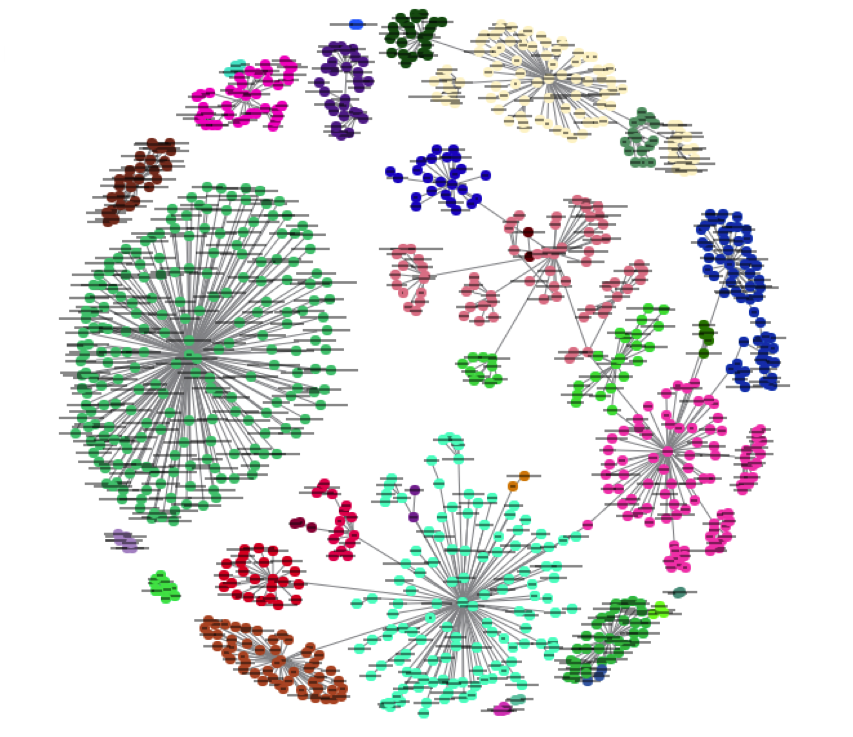#### Machine Learning: kNN AlgorithmAnalytics Vidhya

The k-Nearest Neighbors(k-NN) algorithm is simple and easy to implement. It is Supervised Learning technique which can solve both Classification and Regression problems. So, the first question which…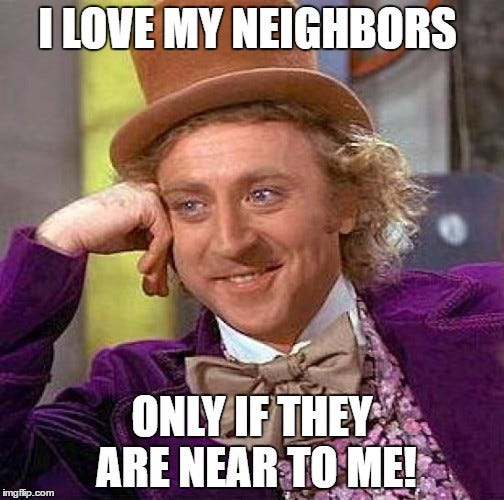#### k-nearest neighboursAnalytics Vidhya

KNN is a simple and intuitive Machine Learning Algorithm. It can be used for both classification and regression. It is a sort of Supervised Learning where we get both x and y. KNN is Non-Parametric…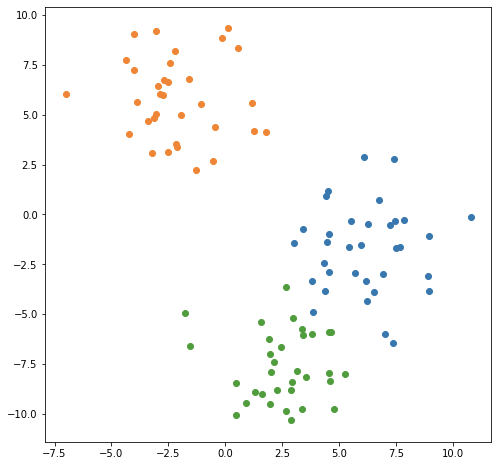#### The Basics: KNN for classification and regressionTowards Data Science

Data science or applied statistics courses typically start with linear models, but in its way, K-nearest neighbors is probably the simplest widely used model conceptually. KNN models are really just…

Read more at Towards Data Science#### K-Nearest Neighbors (KNN)Python in Plain English

Implementation and evaluation of KNN model in python Creating a model to make predictions based on fresh data or forecast future occurrences based on unobserved data is the ultimate objective of a ma...

Read more at Python in Plain English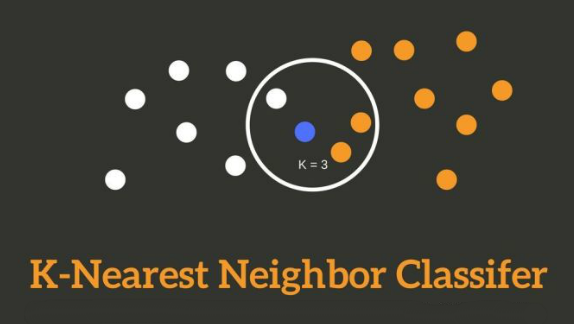#### K-Nearest Neighbors (KNN)Analytics Vidhya

In this Blog I will be writing about a very famous supervised learning algorithm, that is, k-nearest neighbors or in short KNN. Here I will explain about what is KNN algorithm, Industrial uses of KNN…...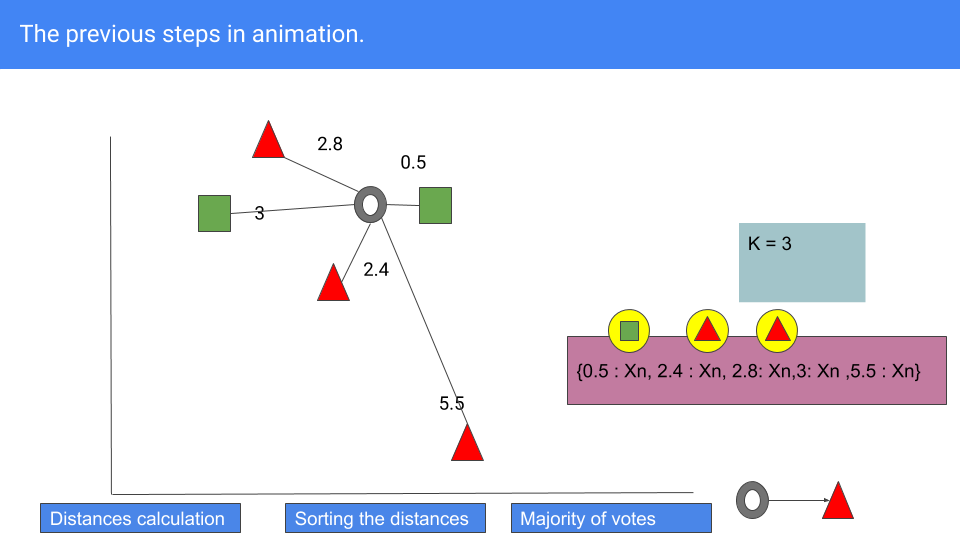#### Day two in Machine Learning: KNNAnalytics Vidhya

Learn the basic of KNN algorithm for Machine Learning and implement the code from scratch in Python.#### A Quick Little Lesson on KNNTowards Data Science

As the title says, here is a quick little lesson on how to construct a simple KNN model in SciKit-Learn. I will be using this dataset. It contains information on students’ academic performance…

Read more at Towards Data Science#### Let’s Make a KNN Classifier from ScratchTowards Data Science

Coding out the entire machine algorithms from scratch can be a tedious process. That’s why we have libraries. The other reason is, our own implementation most likely cannot compete with out of the…

Read more at Towards Data Science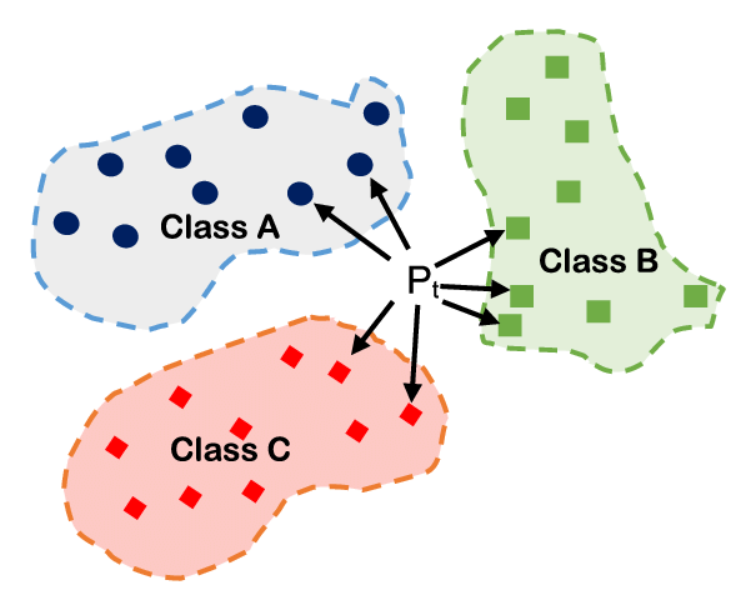#### K-Nearest Neighbors (KNN) for Machine LearningAnalytics Vidhya

If you are familiar with machine learning and the basic algorithms used in the field, then you may have heard of the algorithm k-nearest neighbors or KNN. One of the more simple techniques used in…#### 5 Steps to Build a KNN ClassifierTowards AI

The k-nearest neighbor algorithm is applied to different classification and regression problems. The closest k training samples are used to predict the class of new input data, i.e., the most similar…...

#### KNN: K-Nearest NeighboursLevel Up Coding

To start with, K-NN is classified as a supervised machine learning algorithm. For those who want to understand supervised and unsupervised machine learning algorithm, here is a link for the same. In…

Read more at Level Up Coding#### KNN(K_Nearest Neighbors)Analytics Vidhya

KNN is a supervised machine learning algorithm (a dataset which has been labelled) is used for binary as well as multi class classification problem especially in the field of economic forecasting…#### Use Jax to Create a KNN ClassifierPython in Plain English

The k-nearest neighbours algorithm, also known as KNN or k-NN, is a non-parametric, supervised learning classifier, which uses proximity to make classifications or predictions about the grouping of an...

Read more at Python in Plain English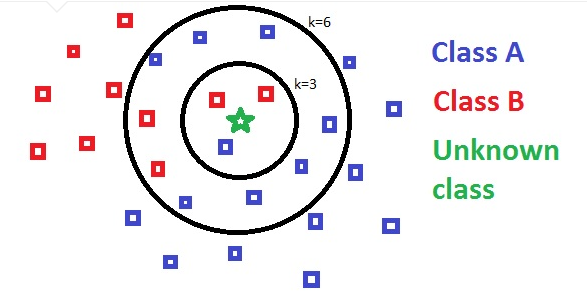#### KNN using scikit-learnTowards Data Science

KNN (K-Nearest Neighbor) is a simple supervised classification algorithm we can use to assign a class to new data point. It can be used for regression as well, KNN does not make any assumptions on…

Read more at Towards Data Science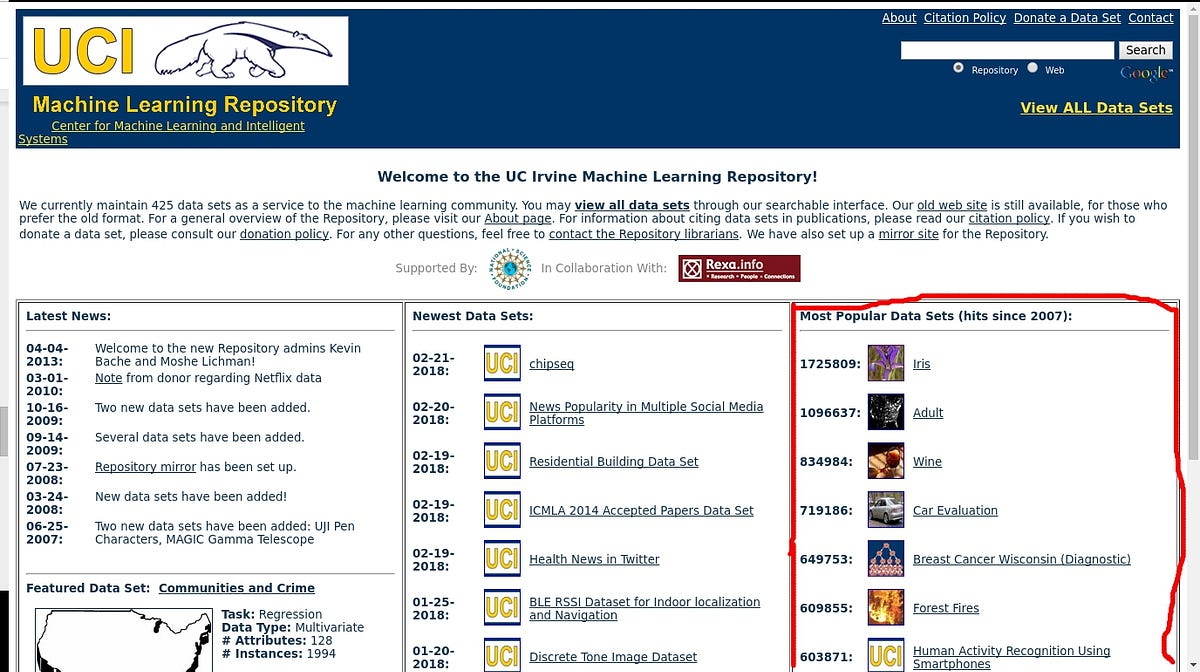#### KNN (K-Nearest Neighbors) #2Towards Data Science

If you have not read yet, or don’t know how KNN works, I suggest you read this post before you continue reading this, so you will find the algorithm’s explanation without codes. Let’s start! First…

Read more at Towards Data Science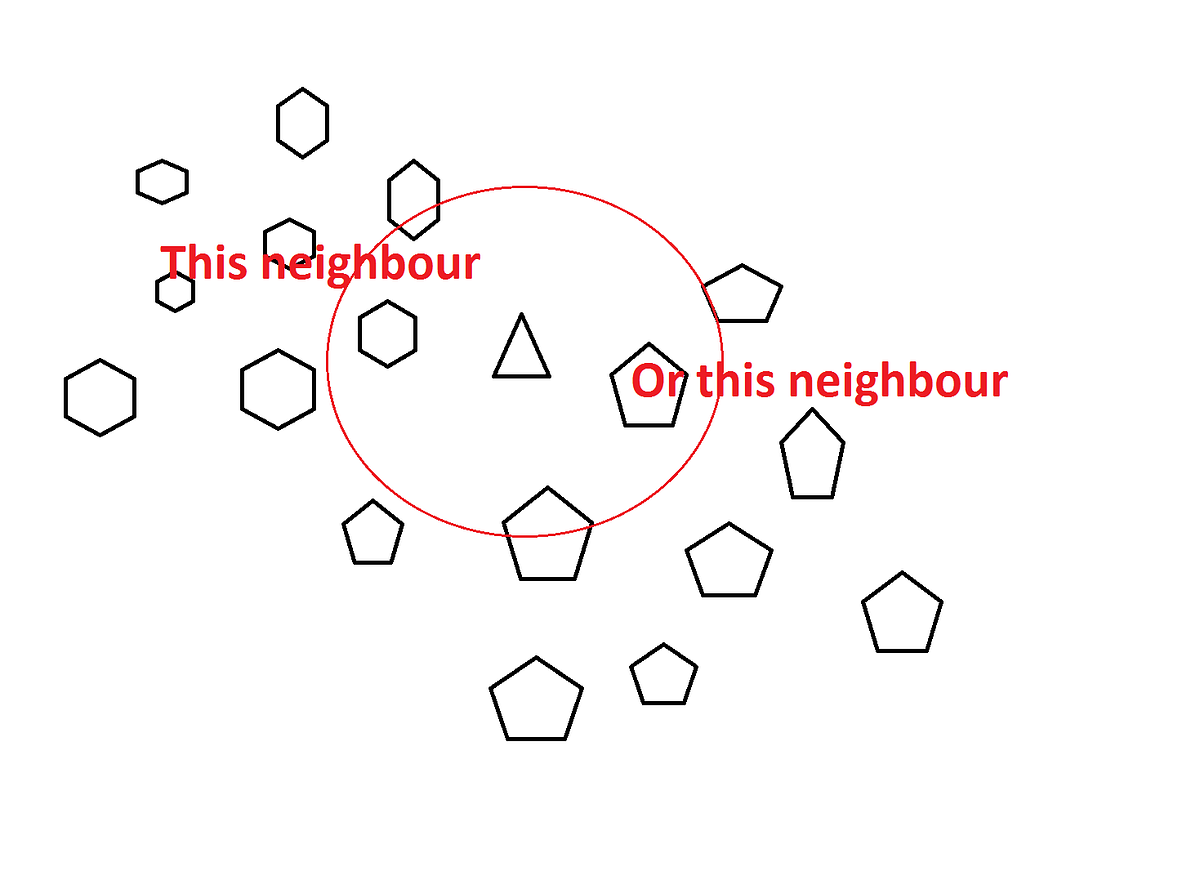#### KNN Algorithm: What?When?Why?How?Towards Data Science

KNN: K Nearest Neighbor is one of the fundamental algorithms in machine learning. Machine learning models use a set of input values to predict output values. KNN is one of the simplest forms of…

Read more at Towards Data Science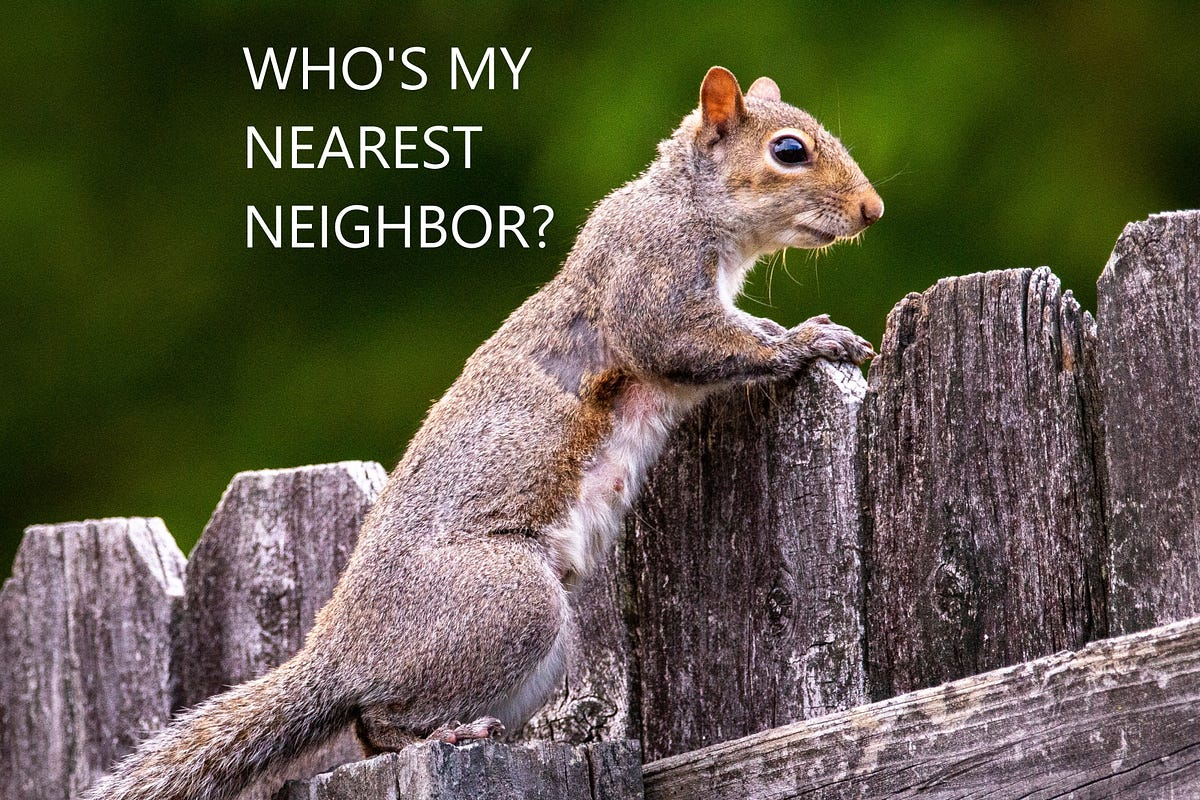#### K-Nearest Neighbors (KNN) algorithmTowards Data Science

K nearest neighbors (KNN) is a supervised machine learning algorithm. A supervised machine learning algorithm’s goal is to learn a function such that f(X) = Y where X is the input, and Y is the…

Read more at Towards Data Science#### k-nearest-neighbor (KNN) algorithmTowards Data Science

Data science is hot and it seems that everybody is working on some sort of project involving the latest state-of-the-art (SOTA) algorithm. Of course with good reason as in many cases we can use data…

Read more at Towards Data Science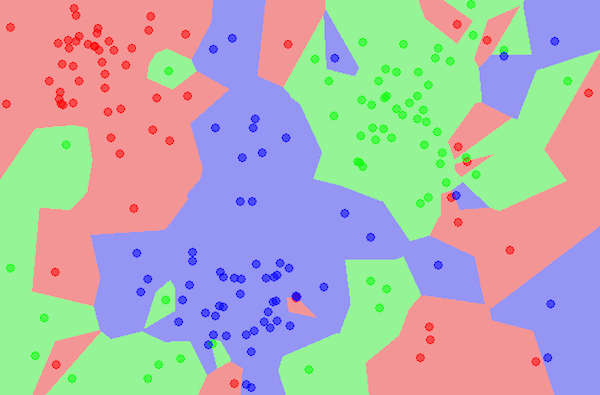#### Fundamentals Of Machine Learning Part 5- KNN(K Nearest Neighbors)Analytics Vidhya

In this article we are going to talk about a very famous machine learning algorithm that is used for classification as well as for regression. KNN algorithm works on the assumption that data having…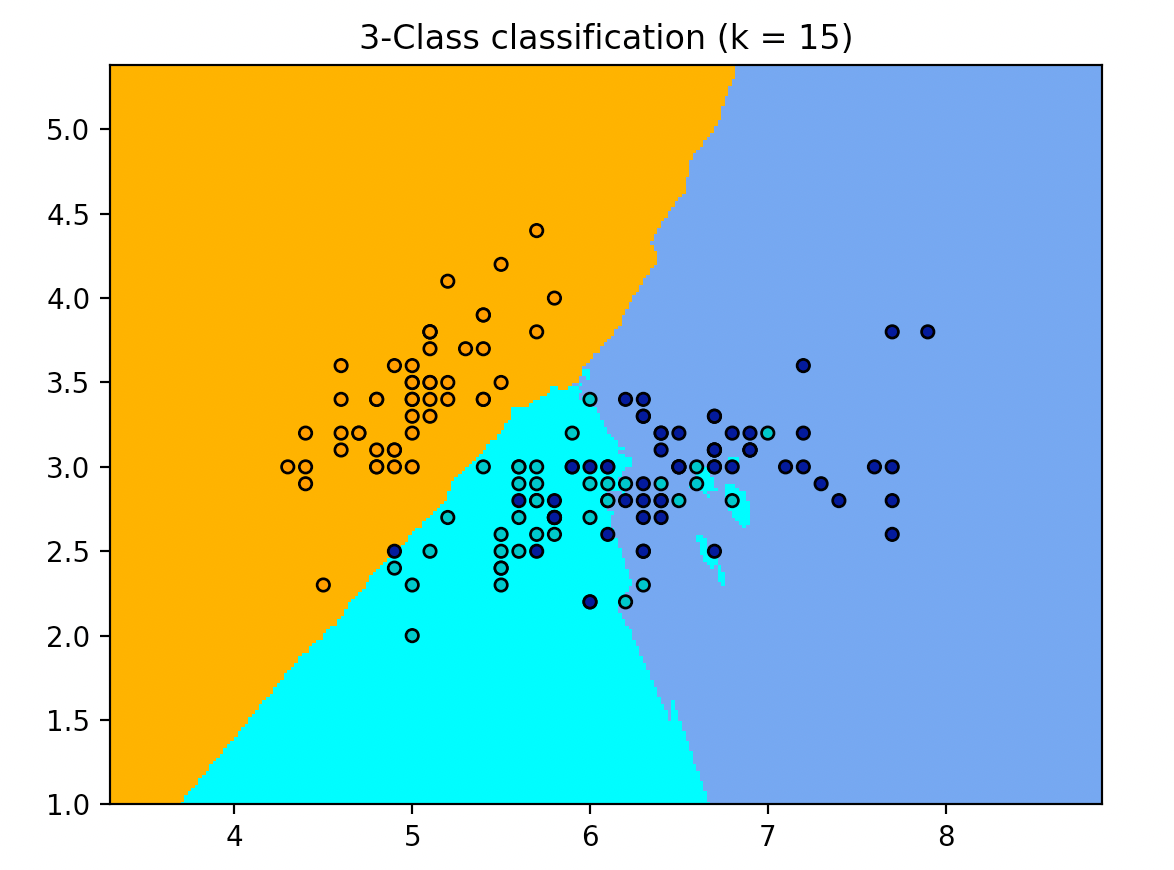Analytics Vidhya

KNN stands for K nearest neighbors and it is supervised learning algorithm. As a name suggests, this algorithm depends upon the observation’s nearest neighbour and their classes.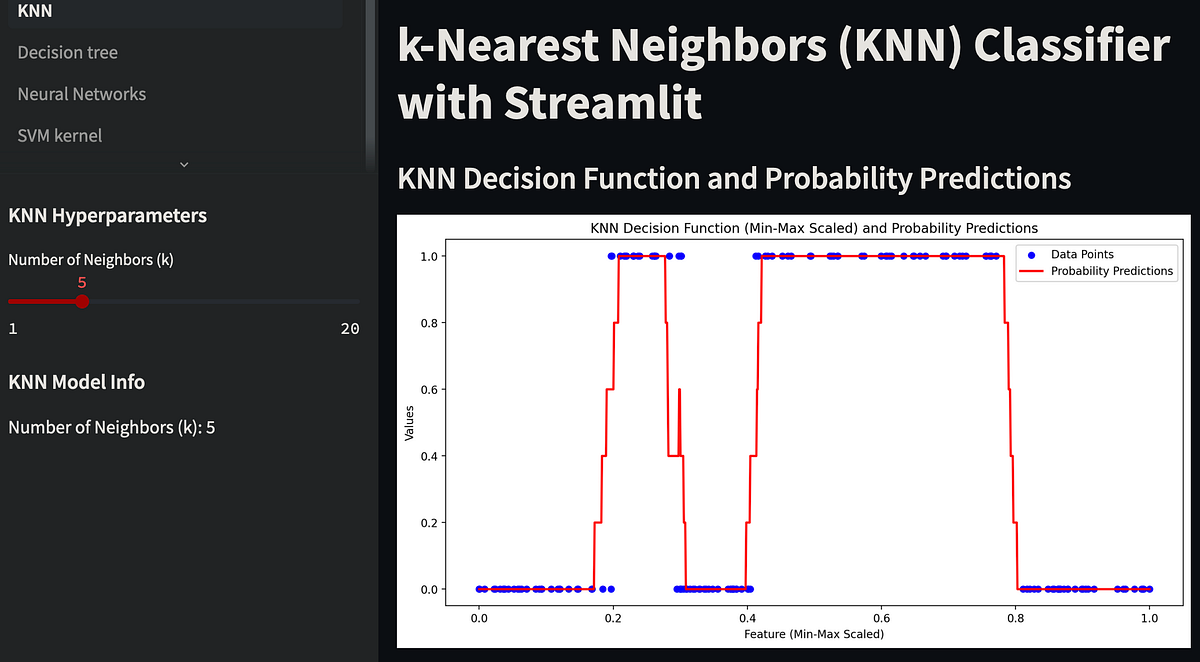#### Understanding K-Nearest Neighbors (KNN) Classification with VisualizationsPython in Plain English

The Basics of KNN Classification At the heart of the KNN algorithm is the idea that objects are more likely to be similar if they are close to each other. In the context of classification, KNN determi...

Read more at Python in Plain English#### Homo sapiens — the KNN classifierTowards Data Science

Homo sapiens — the KNN classifier How our decisions are governed by the KNN approach Photo by Sylas Boesten on Unsplash The K-nearest neighbours (KNN) method is probably the most intuitive classifier...

Read more at Towards Data Science